Free Printable Worksheets for Second Grade

## Subtraction Worksheet – 3 digit subtraction without regrouping (Set B)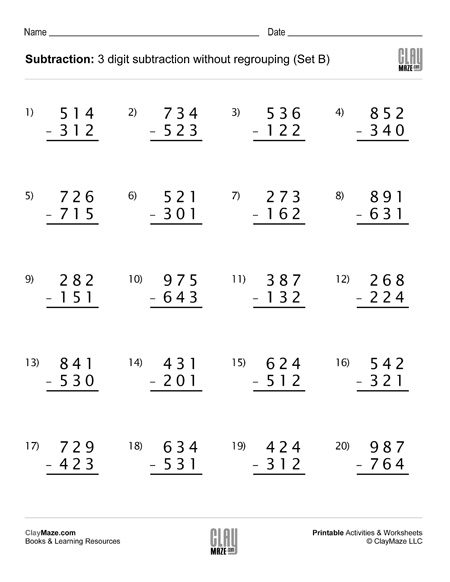3 digit subtraction worksheet – no regrouping, no borrowing. Set of 20 subtraction problems for lots of math practice....

## Children’s Puzzle: Brain / Circle Shaped MazeEnjoy this brain / circle-shaped maze and remember that the second page of the PDF is the solution, so don’t peek! Holding a child’s visual attention can be a difficult thing. Their eyes and focus ...

## Children’s Puzzle: Plus Sign Maze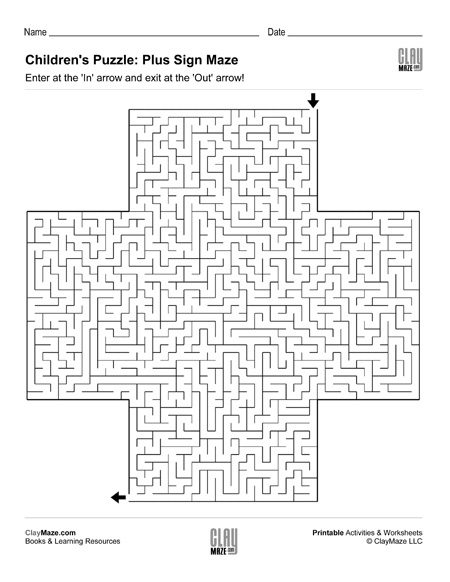Enjoy this plus-sign shaped maze and please note the second page of the PDF is the solution so remember not to peek!  We have a variety of printable mazes, puzzles and other fun worksheets...

## Homonym / Homophone Science Facts, Worksheet 1Due to the advent of computers with grammar and spell check ability, many of us might think our children may not need to focus on those skills quite as much as we did when we were younger. Unfortun...

## Homonym / Homophone Science Facts, Worksheet 2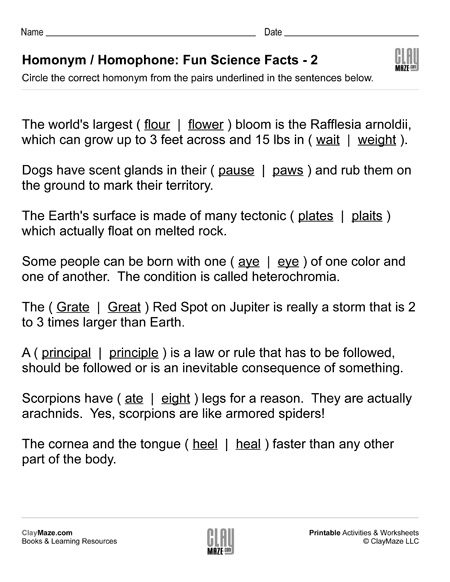Homophones are words that sound the same but have different spellings and meanings. We all make a mistake from time-to-time and interchange a ‘too’ for a ‘two’ or a ‘there’ for a ‘their’, but there...

## Homonym / Homophone Science Facts, Worksheet 3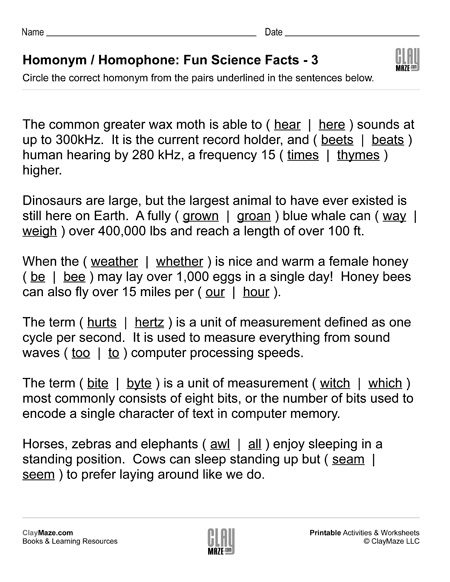There are many words that sound the same but have different meanings and spellings.  Those words are called homophones and interchanging them in our writings can wreak havoc on an otherwise perfect...

## Fraction Addition with Same Denominators (Set 1)Math worksheet – fractions with the same denominators. Write the fractions below the pictures and add them. Color the sum in the picture and write the correct fraction under it....

## Fraction Addition with Same Denominators (Set 2)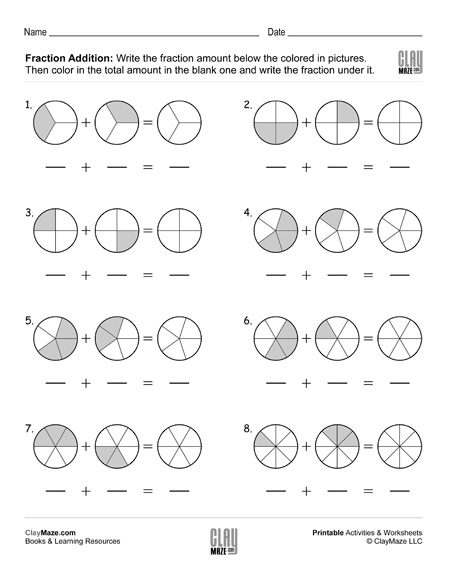Fractions worksheet with same denominators. Write the fraction amounts under the pictures. Color the total fraction amount in the blank picture and write the sum under it....

## Fraction Addition with Same Denominators (Set 3)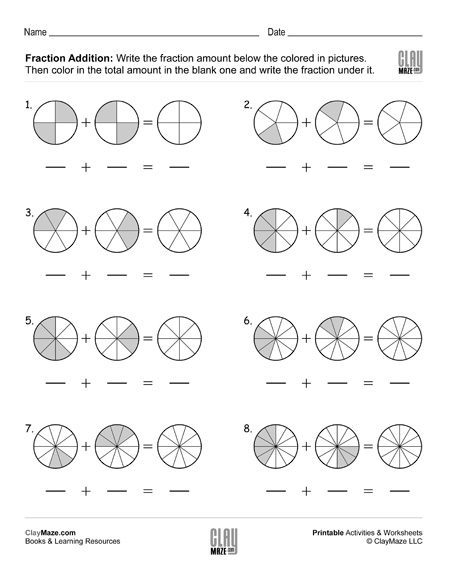Adding fractions with common denominator worksheet. Add the fractions and color the sum in the picture....

## Fraction Addition with Same Denominators (Set 4)Fraction worksheet – Write the fraction amount under he picture. Then color in the total fraction amount and write the sum in the blank below it....

## Fraction Addition with Same Denominators (Set 5)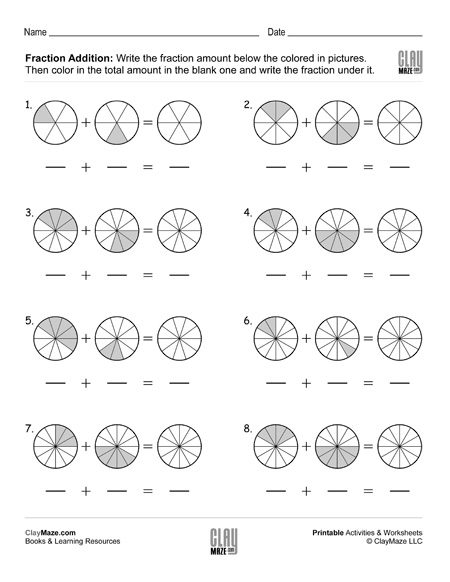Fraction math: addition – Write the fraction amounts below the pictures. Color in the total amount in the blank and write the amount below it.  ...

## Fraction Subtraction with Same Denominators (Set 1)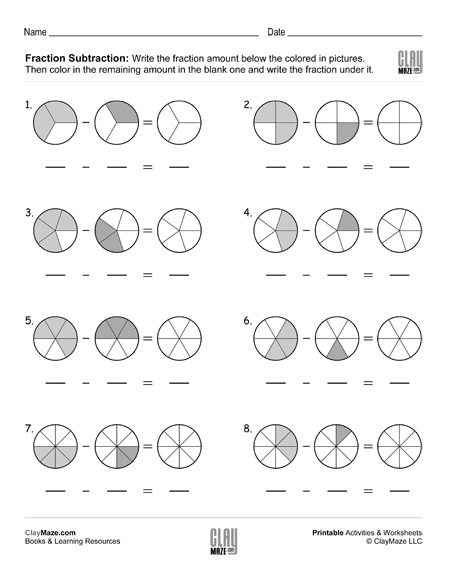Subtracting fractions worksheet – Write the fraction amount below the picture. Subtract the fractions and color in the remaining amount in the picture....

## Fraction Subtraction with Same Denominators (Set 2)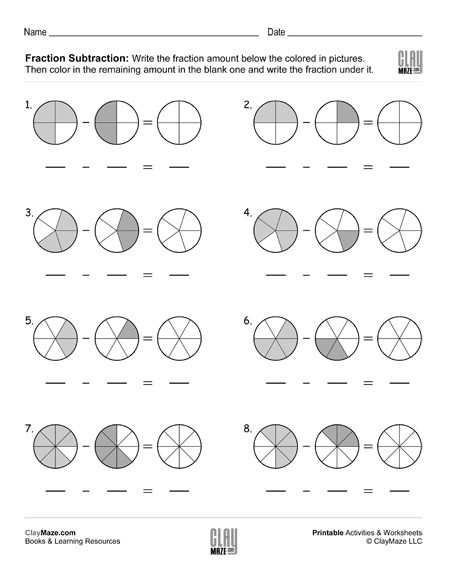Fraction subtraction with common denominators – write the fractions, then subtract them using the pictures and color in the correct amount....

## Fraction Subtraction with Same Denominators (Set 3)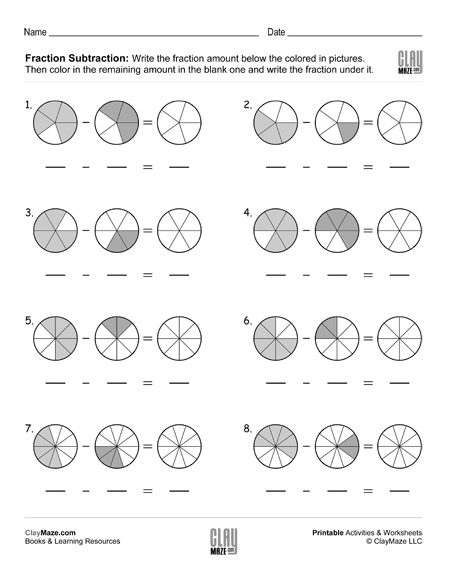Subtracting fractions free, printable worksheet – Write the fractions, subtract them and color the remaining amount....

## Fraction Subtraction with Same Denominators (Set 4)Fraction subtraction with common denominators worksheet – Write the correct fraction amount under each picture. Subtract the fractions and color the fractional amount in the blank picture....